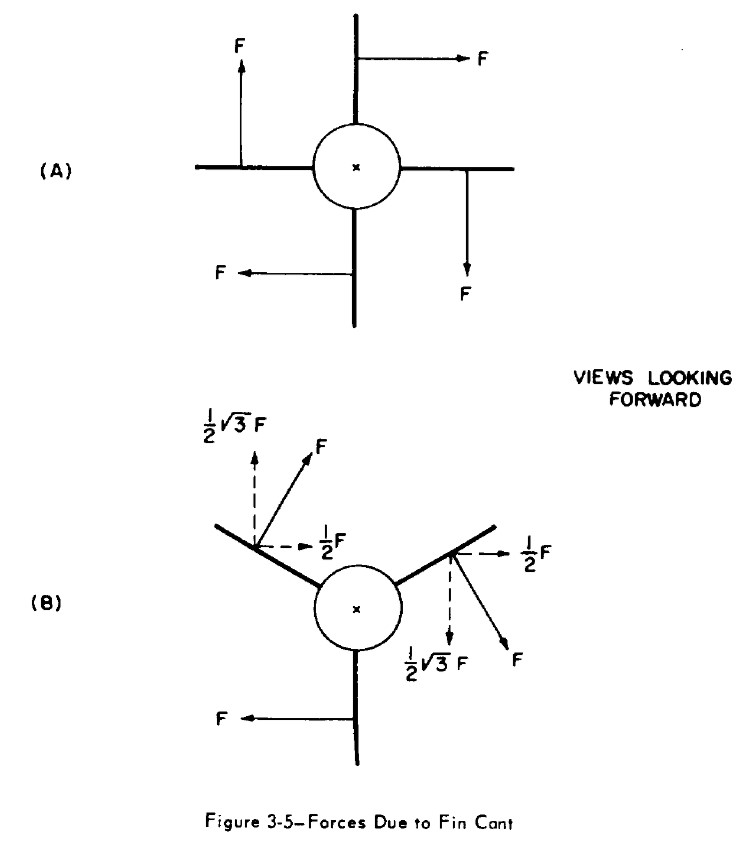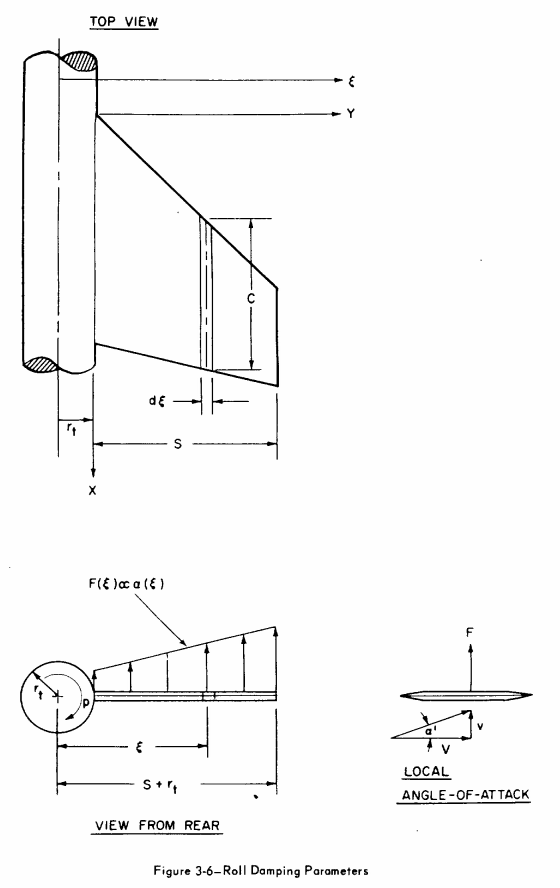# Roll equations for high-powered rockets#

Author:

Bruno Abdulklech Sorban,

Author:

Mateus Stano Junqueira

Date:

February 2022

## Nomenclature#

• $$A_{r}$$ - Reference area

• $$(C_{N\alpha})_{0}$$ - Normal force coefficient derivative of a 2D airfoil

• $$(C_{N\alpha})_{1}$$ - Normal force coefficient derivative of one fin

• $$C_r$$ - Root chord

• $$C_t$$ - Tip Chord

• $$F$$ - Force

• $$L_{r}$$ - Reference length, rocket diameter

• $$M_{roll}$$ - Roll moment

• $$M_{f}$$ - Roll forcing moment

• $$M_{d}$$ - Roll damping moment

• $$N$$ - Number of fins

• $$\overline{q}$$ - Dynamic pressure

• $$r_t$$ - Reference radius at fins position

• $$s$$ - Span

• $$v_{0}$$ - Rocket speed in relation to the wind

• $$\omega$$ - Angular velocity

• $$Y_{MA}$$ - Spanwise location of mean aerodynamic chord measured from the root chord

• $$\delta$$ - Fin cant angle

• $$\xi$$ - Distance to rotation axis

• $$\rho$$ - Ambient density

• $$C_{lf}$$ - Roll moment lift coefficient

• $$C_{lf\delta}$$ - Roll moment lift coefficient derivative

• $$C_{ld}$$ - Roll moment damping coefficient

• $$C_{ld\omega}$$ - Roll moment damping coefficient derivative

## Introduction#

Calculating the rotational movement of a high powered rocket entails calculating the Roll Moment. Here all formulas and consideration for the implementation of the Roll Moment in RocketPy are shown and explained.

The main cause for a rocket roll movement are certain asymmetries in its construction. The most noteworthy of this possible asymmetries is the fin cant angle ($$\delta$$), which can be seen in Figure 1, and will be considered for the calculations.## Coefficient derivatives#

According to the equation formulated by [Barrowman], the rotational moment around the rockets axis is governed by two main forces: one that causes the rolling movement and one that damps the movement. Each of these forces generates its own moment, the forcing moment $$M_{f}$$ and the damping moment $$M_d$$. The final roll moment can then be calculated with:

$M_{roll} = M_{forcing} - M_{damping}$

### Roll Forcing#

Roll forcing is the moment that causes the rocket to rotate around its axis. The calculations for this moment assumes $$\omega = 0$$ e $$\delta \neq 0$$.

Due to the symmetry of the fins - as can be seen in Figure 2 - the forces cancel each other out so that the resulting force $$F_{R}$$ is equal to zero. However, the resulting moment $$M_{R} \neq 0$$, that is, it constitutes a gyroscope binary.According to [Barrowman], equation (3-31), the roll forcing moment is given by:

$M_f = N (Y_{MA} + r_t)(C_{N\alpha})_1\delta \overline{q}A_r$

The author also defined the roll forcing moment coefficient as:

$C_{lf} = \frac{M_f}{\overline{q} A_r L_r}$

The letter “$$f$$” has been added to the name to differentiate Forcing from Damping. Note the similarity with the definition of drag coefficient ($$C_{d} = \frac{2F_{Drag}}{\rho V^2 A_{ref}}$$). Finally, you can also calculate $$C_{lf}$$ as:

$C_{lf} = \frac{N(Y_{MA} + r_t)(C_{N\alpha})_1 \delta}{L_r}$

And its derivative relative to the cant angle as:

$C_{lf\delta} = \frac{\partial C_{lf}}{\partial \delta}$
$C_{lf\delta} = \frac{N (Y_{MA} + r_t) (C_{N\alpha})_1 }{L_r}$

The forcing moment is then calculated with:

$M_f = \overline{q} \,\, A_r L_r \,\, C_{lf\delta}\,\, \delta$

or

$M_f = \frac{1}{2} \rho v_0^2 \,\, A_r L_r \,\, C_{lf\delta}\,\, \delta$

### Roll Damping#

While roll forcing causes the rotation movement, the roll damping force is what counteracts this movement. It is a force that scales with angular velocity and acts in the opposite direction. $$\omega \neq 0$$ and $$\delta = 0$$ are assumed.

It is defined in the same way as roll forcing:

(1)#$C_{ld} = \frac{M_d}{\overline{q} A_r L_r}$

From [Barrowman], the roll damping moment depends on the angle of attack of the tangential velocity of the fin panel at a certain span wise position $$\xi$$, as can be seen in Figure 3.The damping moment at $$\xi$$ is:

$d M = \xi F(\xi)$

Where $$F(\xi)$$ is the force generated at the span wise position $$\xi$$ and its given by:

$F(\xi) = - C_{N_{\alpha 0}} \, \overline{q} \, a(\xi) \,c(\xi) \, d\xi$

$$a(\xi)$$ is the local angle of attack at $$\xi$$ and is given by:

$a(\xi) = \tan^{-1}(\frac{\omega \, \xi}{v_0} )$

An approximation that is valid when $$v_0 >> \omega \, \xi$$ is made

$a(\xi) = - \frac{\omega \, \xi}{v_0}$

$$c(\xi)$$ is the cord length at the span wise $$\xi$$ and is calculated differently for each fin shape. The damping moment can then be written as:

$dM = - \frac{C_{N_{\alpha 0}} \, \overline{q} \, \omega}{v_0} \,\, c(\xi) \,\xi^2 \, d\xi$

From  we know that:

$d C_{ld} = \frac{C_{N_{\alpha 0}} \, \, \omega}{v_0 \ A_r \, L_r} \,\, c(\xi) \,\xi^2 \, d\xi$

Integrating over the exposed fin geometry:

$C_{ld} = \frac{C_{N_{\alpha 0}} \, \, \omega}{v_0 \ A_r \, L_r} \,\, \int_{r_t}^{s + r_t} c(\xi) \, \xi^2 \, d\xi$

The initial hypothesis assumes that, for the roll damping calculation, the deflection is $$\delta = 0$$. This implies a larger cross-sectional area than is actually acting against the movement (analogous to flow passing through a surface). As a result, the term $$\cos(\delta)$$ was added to the original formulation:

$C_{ld} = \frac{C_{N_{\alpha 0}} \, \, \omega}{v_0 \ A_r \, L_r} \, \cos(\delta) \, \int_{r_t}^{s + r_t} c(\xi) \, \xi^2 \, d\xi$

The roll damping coefficient derivative can then be defined as:

$C_{ld\omega} = \frac{\partial C_{ld}}{\partial (\frac{\omega L_{r}}{2v_0})}$
$C_{ld\omega} = \frac{2 \ C_{N_{\alpha 0}} \, }{A_r \, L_r^2} \, \cos(\delta) \, \int_{r_t}^{s + r_t} c(\xi) \, \xi^2 \,d\xi$

Finally, $$C_{N_{\alpha 0}}$$ must be corrected for three dimensional effects:

$C_{ld\omega} = \frac{2 \ N\ \Bigl(C_{N_{\alpha }}\Bigr)_1 \, }{A_r \, L_r^2} \, \cos(\delta) \, \int_{r_t}^{s + r_t} c(\xi) \, \xi^2 \,d\xi$

The values of the definite integral can be calculated for each specific fin shape. For trapezoidal fins:

$c(\xi) = C_r \left[1 - \frac{1 - \frac{C_t}{C_r}}{s}(\xi - r_t)\right]$
$\int_{r_t}^{s + r_t} c(\xi) \, \xi^2 \, d\xi = \frac{s}{12} \left[(C_r + 3C_t) s^2 + 4(C_r + 2C_t)s r_t + 6(C_r+C_t)r_t^2 \right]$

And for ellipsoidal fins:

$c(\xi) = C_r \sqrt{1 - \Bigl(\frac{\xi - r_t}{s}\Bigr)^2}$
$\int_{r_t}^{s + r_t} c(\xi) \, \xi^2 \, d\xi = C_r\, s\ \frac{ \Bigl(3\pi s^2 + 32 r_t s + 12 \pi r_t^2 \Bigr)}{48}$

The damping moment is finally:

$M_d = \frac{1}{2} \rho v_0^2 \ A_{ref} \, L_{ref} \ C_{ld\omega} \, \frac{\omega L_{ref}}{2 v_0}$

## Interference Coefficients#

In [Barrowman] some fin-body interference factor are calculated. These factors are also implemented in the lift coefficient calculations.

For fins with canted angle:

$\begin{split}K_f &=\frac{1}{\pi^{2}}\left[\frac{\pi^2}{4}\frac{(\tau+1)^{2}}{\tau^2}+\frac{\pi\Bigl(\tau^{2}+1\Bigr)^{2}}{\tau^{2}(\tau-1)^{2}} \sin ^{-1}\Bigl(\frac{\tau^{2}-1}{\tau^{2}+1}\Bigr)-\frac{2 \pi(\tau+1)}{\tau(\tau-1)}\right.\\ &+\frac{\Bigl(\tau^{2}+1\Bigr)^{2}}{\tau^{2}(\tau-1)^{2}}\Bigl(\sin ^{-1} \frac{\tau^{2}-1}{\tau^{2}+1}\Bigr)^{2}-\frac{4(\tau+1)}{\tau(\tau-1)} \sin ^{-1}\Bigl(\frac{\tau^{2}-1}{\tau^{2}+1}\Bigr) \\ &\left.+\frac{8}{(\tau-1)^{2}} \ln \Bigl(\frac{\tau^{2}+1}{2 \tau}\Bigr)\right]\end{split}$

For the damping moment lift coefficient derivative:

$K_d=1+\frac{\frac{\tau-\lambda}{\tau}-\frac{1-\lambda}{\tau-1} \ln \tau}{\frac{(\tau+1)(\tau-\lambda)}{2}-\frac{(1-\lambda)\Bigl(\tau^{3}-1\Bigr)}{3(\tau-1)}}$
Where $$\tau = \frac{s + r_t}{r_t}$$ and $$\lambda = \frac{C_t}{C_r}$$. The final lift coefficients are:
$(C_{lf\delta})_{K_{f}} = K_{f} \cdot C_{lf\delta}$
$(C_{ld\omega})_{K_{d}} = K_{d} \cdot C_{ld\omega}$

$f_{eq} = \frac{\omega}{2\pi} = \frac{A_{ref}\beta \overline{Y_t} (C_{N\alpha})_1 }{4\pi^2 \sum_{i} c_i \xi^2 \Delta \xi} \, \delta V_0$
The auxiliary value $$\beta$$ is defined as: $$\beta = \sqrt{|1-M|}$$, where M is the speed of the rocket in Mach.